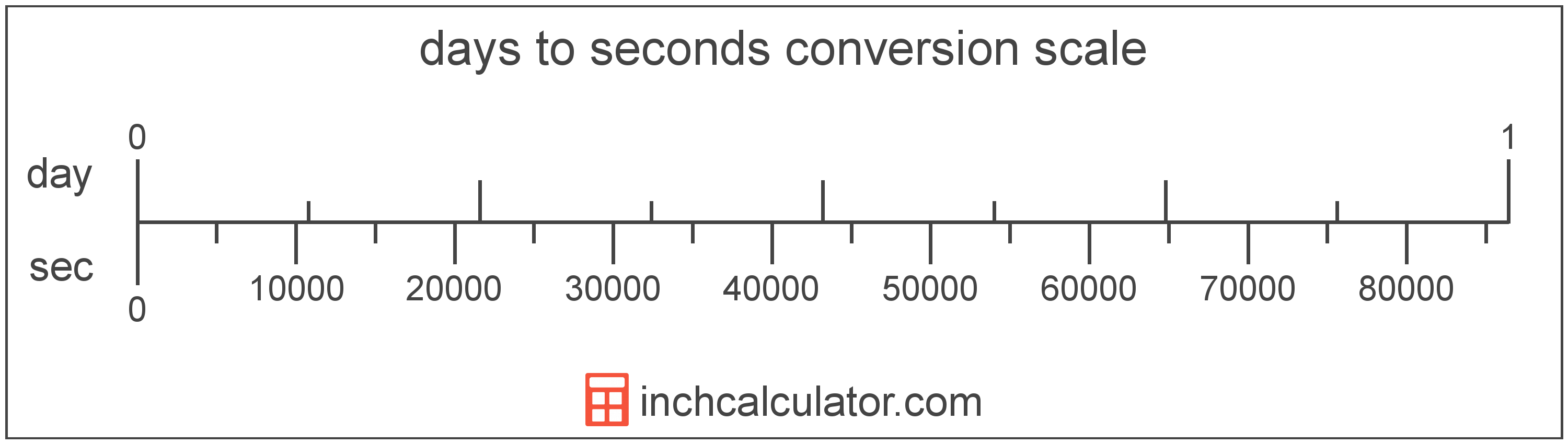# Days to Seconds Conversion

Enter the time in days below to get the value converted to seconds.

Results in Seconds:1 day = 86,400 sec
Do you want to convert seconds to days?

## How to Convert Days to SecondsTo convert a day measurement to a second measurement, multiply the time by the conversion ratio.

Since one day is equal to 86,400 seconds, you can use this simple formula to convert:

seconds = days × 86,400

The time in seconds is equal to the days multiplied by 86,400.

For example, here's how to convert 5 days to seconds using the formula above.
5 day = (5 × 86,400) = 432,000 sec

### How Many Seconds are in a Day?

There are 86,400 seconds in a day, which is why we use this value in the formula above.

1 day = 86,400 sec

## Days

One day is the time it takes for the Earth to complete a single rotation around its axis in relation to the Sun. There are 24 hours in a day.

The day is an SI accepted unit for time for use with the metric system. Days can be abbreviated as day; for example, 1 day can be written as 1 day.

## Seconds

The second has historically been defined as 1/60 of a minute or 1/86,400 of a day. According the to SI definition, the second is the duration of 9,192,631,770 periods of the radiation corresponding to the transition between the two hyperfine levels of the unperturbed ground state of the caesium 133 atom.

The second is the SI base unit for time in the metric system. Seconds can be abbreviated as sec; for example, 1 second can be written as 1 sec.

## Day to Second Conversion Table

Day measurements converted to seconds
Days Seconds
0.0001 day 8.64 sec
0.0002 day 17.28 sec
0.0003 day 25.92 sec
0.0004 day 34.56 sec
0.0005 day 43.2 sec
0.0006 day 51.84 sec
0.0007 day 60.48 sec
0.0008 day 69.12 sec
0.0009 day 77.76 sec
0.001 day 86.4 sec
0.002 day 172.8 sec
0.003 day 259.2 sec
0.004 day 345.6 sec
0.005 day 432 sec
0.006 day 518.4 sec
0.007 day 604.8 sec
0.008 day 691.2 sec
0.009 day 777.6 sec
0.01 day 864 sec
0.02 day 1,728 sec
0.03 day 2,592 sec
0.04 day 3,456 sec
0.05 day 4,320 sec
0.06 day 5,184 sec
0.07 day 6,048 sec
0.08 day 6,912 sec
0.09 day 7,776 sec
0.1 day 8,640 sec
0.2 day 17,280 sec
0.3 day 25,920 sec
0.4 day 34,560 sec
0.5 day 43,200 sec
0.6 day 51,840 sec
0.7 day 60,480 sec
0.8 day 69,120 sec
0.9 day 77,760 sec
1 day 86,400 sec

## References

1. International Bureau of Weights and Measures, The International System of Units, 9th Edition, 2019, https://www.bipm.org/utils/common/pdf/si-brochure/SI-Brochure-9.pdf